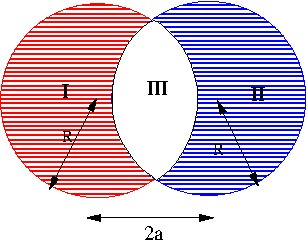# [YMP/EM-02003] Dipole Moment of Overlapping Spheres

For page specific messages
For page author info
 Two spheres each of radius $R$ are placed so that they partially overlap. The charge densities in the overlap region is zero. Outside the overlap region but inside the spheres the charge densities in the two non overlapping   regions are $+\rho$ and $-\rho$, respectively, as shown in figure. The charge density in the overlap region will be zero. The separation between the centers of the spheres is $D$.1. Guess the value of the dipole moment?
2. Compute the dipole moment of the system and check the correctness of the expression you guessed.
3. How will the dipole moment change if the two spheres equal and opposite total charges but have different radii $$a,b, a \ne b$$.
1. Let the centres of the spheres be $$\vec a$$ and $$\vec b$$. We can assume that the two spheres have charge densities $$\rho$$ and $$-\rho$$ inside their respective volumes. Then the charge density in the overlap region will be zero as is specified in the problem. Thus the problem reduces to finding dipole moment of a uniformly charged sphere of radius $$R$$ and centre at $$\vec r_0$$. This distribution will have dipole moment equal to $$Q\vec r_0$$. It then follows that the dipole moment of the given system will be $\vec p = Q(\vec a-\vec b)$
2. The above result is easily derived by setting up the integral for the dipole moment \begin{eqnarray} \vec p &=& \iiint_{|\vec r-\vec r_0|=R} (\vec r)\rho(\vec r)d^3r \\ &=&\rho \iiint_{|\vec r-\vec r_0|=R} \vec r d^3r \end{eqnarray} Shifting the origin to the point $$r_0$$, define new position as $$\vec u= \vec r-\vec r_0$$, we get dipole moment \begin{eqnarray} \vec p &=&\rho \iiint_{|\vec u|=R} [\vec u + \vec r_0] d^3u = \rho \times \frac{4\pi}{3} R^3 \vec r_0. \end{eqnarray} The first integral vanishes and the second integral is just the volume of the sphere. If $$Q$$ is the total charge and $$V$$ is the volume of the sphere then $$Q=\rho V$$. \begin{eqnarray} \vec p &=& \rho \iiint_{|\vec u|=R} [\vec u] d^3u + \vec r_0] \rho \iiint_{|\vec u|=R} d^u\\ &=& \rho\Big\{\frac{4\pi}{3} R^3 \Big\}\ \vec r_0 \\ &=& Q \vec r_0. \end{eqnarray} where $$Q$$ is the total charge on the sphere. Therefore the required dipole moment of the system of two spheres is just the sum of the individual dipole moments. \begin{equation} \vec p = Q(\vec a -\vec b) = Q\vec D. \end{equation}
3. The answer does not change if the radii are different, and if the total charges are equal and opposite.

n
0

X